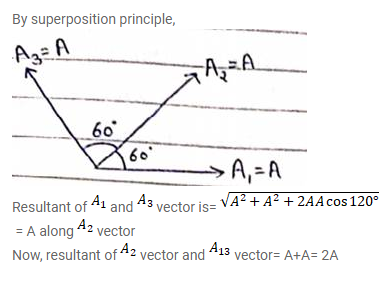# Three simple harmonic motions of equal amplitudes A and equal

Question:

Three simple harmonic motions of equal amplitudes A and equal time periods in the same direction combine. The phase of the second motion is $60^{\circ}$ ahead of the second. Find the amplitude of the resultant motion.

Solution: